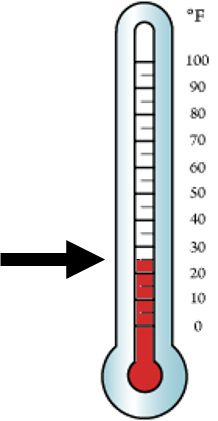1
visibility

What is the temperature shown on the given thermometer?• \$latex 15^\circ F\$

• \$latex 25^\circ F\$

• \$latex 35^\circ F\$

The correct answer is  25°F.

Solution:

First, we find the point where the thermometer changes from red to white.Then, we look at the number to the right.

The temperature is  25°F  as desired.